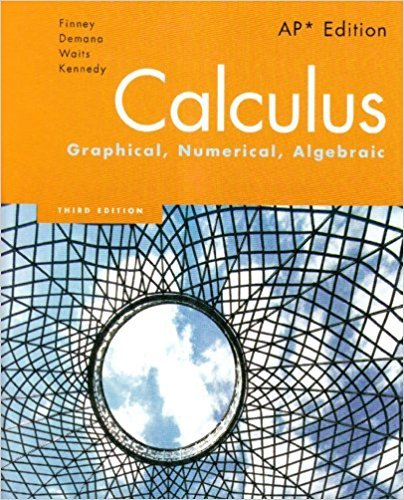×
Get Full Access to Calculus: Graphical, Numerical, Algebraic - 3 Edition - Chapter 7 - Problem 1
Get Full Access to Calculus: Graphical, Numerical, Algebraic - 3 Edition - Chapter 7 - Problem 1

×

# A toy car slides down a ramp and coasts to a stop after 5ISBN: 9780132014083 136

## Solution for problem 1 Chapter 7

Calculus: Graphical, Numerical, Algebraic | 3rd Edition

• Textbook Solutions
• 2901 Step-by-step solutions solved by professors and subject experts
• Get 24/7 help from StudySoup virtual teaching assistantsCalculus: Graphical, Numerical, Algebraic | 3rd Edition

4 5 1 306 Reviews
13
3
Problem 1

A toy car slides down a ramp and coasts to a stop after 5 sec. Its velocity from t ! 0 to t ! 5 is modeled by v!t" ! t2 " 0.2t 3 ft#sec. How far does it travel?

Step-by-Step Solution:
Step 1 of 3

tF rlr lf /\ l(z ^ "------I 1- \,, t X { ---|-' I -z.Y I ,V .( l+ u\ I X 7Y -__--_-- X \ \ x-{+-x lY ,-* +@ ---\/ Lh + r1t+ (t -*7 xt- )*FnJ ezo()& e) 7\ JvU -{) ,t o)fg'*)'-€:;7x

Step 2 of 3

Step 3 of 3

##### ISBN: 9780132014083

The answer to “A toy car slides down a ramp and coasts to a stop after 5 sec. Its velocity from t ! 0 to t ! 5 is modeled by v!t" ! t2 " 0.2t 3 ft#sec. How far does it travel?” is broken down into a number of easy to follow steps, and 40 words. This full solution covers the following key subjects: SEC, ramp, down, far, its. This expansive textbook survival guide covers 7 chapters, and 464 solutions. The full step-by-step solution to problem: 1 from chapter: 7 was answered by , our top Calculus solution expert on 11/14/17, 08:35PM. Calculus: Graphical, Numerical, Algebraic was written by and is associated to the ISBN: 9780132014083. This textbook survival guide was created for the textbook: Calculus: Graphical, Numerical, Algebraic, edition: 3. Since the solution to 1 from 7 chapter was answered, more than 358 students have viewed the full step-by-step answer.

Unlock Textbook Solution